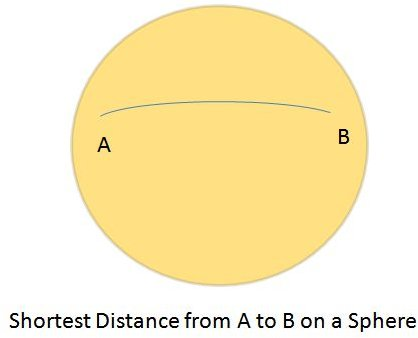# Calculate the Distance Between Two Places on Earth

Today, we have GPS, internet, and other gadgets and tools that will help us calculate distances between one point and another. But imagine the situation nearly two thousand years back. How would you have done it?

Eratosthenes, a greek mathematician, did just that. He came up with a way and found the circumference of the Earth. In other words, the distance between the point where he started (let us call it point A), all the way around the world, back to the point (point A) where he started. He found this using basic mathematics, but with a very interesting approach. Hunderds of years later, it was found that Eratosthenes was very close to the real value that was found using modern tools and equipment.## The Approach Used to Calculate the Distance Between Two Points on Earth

All of us would have learned in our school days that the shortest distance between two points is a straight line. Hence, if you have to find the distance between two points, you will draw a straight line and measure the distance of the straight line between those points. However, this rule applies only for planar surfaces.

Let's take two reference points- point A and point B- separated by a large distance, for easier understanding of the concept.

What will you do if you have to find the distance between two points on Earth? In other words, what approach will you use to calculate the distance between two cities?

Will you stand in a place (point A) and measure the distance to point B by walking in a straight line? You will get the wrong values if you are going to use that approach.That is because the shortest distance on a sphere will appear as a curve on the surface of the sphere and not a straight line. Here is a visual guide on the shortest distance between two points on a sphere.

The straight line approach (in mathematical terms it is called Euclidean Geometry) is much different from the spherical geometry. Given the fact that the Earth is a huge sphere, the approach of using a straight line between two points to calculate the shortest distance will hold true only to a certain distance (ten or twenty kilometers), above which you will be having errors that will affect your further calculations.

## How to Calculate the Distance Between Two Points on Earth

The great circle distance or the orthodromic distance is the shortest distance between two points on a sphere (or the surface of Earth). In order to use this method, you need to have the co-ordinates of point A and point B. (The great circle method is chosen over other methods because it provides a greater accuracy.)

First, convert the latitude and longitude values from decimal degrees to radians. For this divide the values of longitude and latitude of both the points by 180/pi. The value of pi is 22/7. The value of 180/pi is approximately 57.29577951. If you want to calculate the distance between two places in miles, use the value 3,963, which is the radius of Earth. If you want to calculate the distance between two places in kilometers, use the value 6,378.8, which is the radius of Earth.

Find the value of the latitude in radians:

Value of Latitude in Radians, lat = Latitude / (180/pi) OR

Value of Latitude in Radians, lat = Latitude / 57.29577951

Find the value of longitude in radians:

Value of Longitude in Radians, long = Longitude / (180/pi) OR

Value of Longitude in Radians, long = Longitude / 57.29577951

Get the co-ordinates of point A in terms of latitude and longitude. Use the above conversion method to convert the values of latitude and longitude in radians. I will call it as lat1 and long1. Do the same for the co-ordinates of Point B and get lat2 and long2.

Now, to get the distance between point A and point B use the following formula:

Distance, d = 3963.0 * arccos[(sin(lat1) * sin(lat2)) + cos(lat1) * cos(lat2) * cos(long2 – long1)]

The obtained distance, d, is in miles. If you want your value to to be in units of kilometers, multiple d by 1.609344.

d in kilometers = 1.609344 * d in miles

Thus you can have the shortest distance between two places on Earth using the great circle distance approach.

## Tools Used to Find the Distance Between Two Places

Here are a few very reliable tools available on the internet that will help you to easily find the distance between two places.